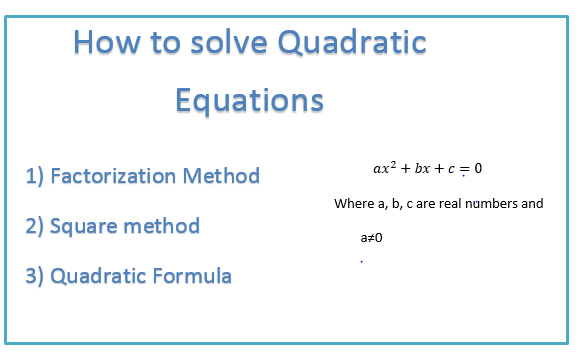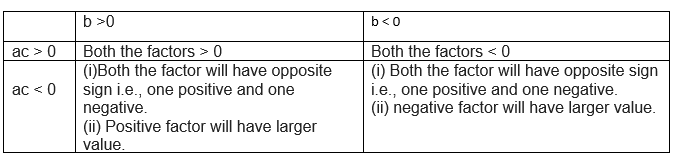# How to solve quadratic equations by factoring

Table of Content

## How to Solve Quadratic equations• There are three ways to solve the quadratic equations.
(a) Factorization method
(b) Square method
• We would be studying detail about each method with some solve examples

In this method of Solving quadratic equations by factoring, we factorize the Quadratic equation by splitting the middle term b

In $ax^2+bx+c=0$

Step 1: Arrange the equation so that coefficient a is positive. If a is negative,multiply each term by -1 to make it positive
Step 2: Find out the product of the coefficient a and c i.e ac.
Step 3: if ac is positive then Find the factor of the product ac such that it adds to form the coefficient b. If b is negative,you can place factors with negative sign and if b is positive, place the factors with positive sign
Step 4: if ac is negative,then Find the factor of the product ac such that it subtract to form the coefficient b. If b is positive, then the larger factor is positive. If b is negative, then the larger factor is negative.
Table below explain the step 3 and 4Step 5:We can write the equation in split form and factorize the equation.
Step 6: Roots of the equation can be find equating the factors to zero

## Solving quadratic equations by factoring Examples

Example -1
$6x^2-x-2=0$
Solution
Step 1 First we need to multiple the coefficient a and c.In this case =6X-2=-12
Step 2 Now the product is negative,so Splitting the middle term so that multiplication is -12 and difference is the coefficient b. Here in this case factor 3, 4 are present,so we can take 3 and -4
$6x^2 +3x-4x-2=0$
$3x( 2x+1) -2(2x+1)=0$
$(3x-2) (2x+1)=0$
step 3 Roots of the equation can be find equating the factors to zero
$3x-2=0$ => $x=\frac {3}{2}$
$2x+1=0$ => $x=\frac {-1}{2}$

Example -2
$x^2 + 7x + 6=0$
Solution
Step 1 First we need to multiple the coefficient a and c.In this case =1X6=6
Step 2 Now the product is positive,so Splitting the middle term so that multiplication is 6 and addition is the coefficient b. Here in this case factor 1, 6 are present,so we can take 1 and 6
$x^2 +6x+x-2=0$
$x( x+1) +6(x+1)=0$
$(x+1) (x+6)=0$
step 3 Roots of the equation can be find equating the factors to zero
$x+1=0$ => $x=-1$
$x+6=0$ => $x=-6$

Practice Questions
• $x^{2} - 13 x + 40 = 0$
• $x^{2} + 2 x - 48 = 0$
• $x^{2} - 8 x + 7 = 0$
• $x^{2} - 1 = 0$
• $x^{2} + 3 x - 54 = 0$
• $x^{2} - 15 x + 56 = 0$
• $x^{2} + 2 x - 3 = 0$
• $x^{2} - 7 x + 6 = 0$
• $x^{2} - 2 x - 63 = 0$
• $x^{2} + x - 42 = 0$

• $x = 5, x = 8$
• $x = -8, x = 6$
• $x = 1, x = 7$
• $x = -1, x = 1$
• $x = -9, x = 6$
• $x = 7, x = 8$
• $x = -3, x = 1$
• $x = 1, x = 6$
• $x = -7, x = 9$
• $x = -7, x = 6$Go back to Class 10 Main Page using below links

### Practice Question

Question 1 What is $1 - \sqrt {3}$ ?
A) Non terminating repeating
B) Non terminating non repeating
C) Terminating
D) None of the above
Question 2 The volume of the largest right circular cone that can be cut out from a cube of edge 4.2 cm is?
A) 19.4 cm3
B) 12 cm3
C) 78.6 cm3
D) 58.2 cm3
Question 3 The sum of the first three terms of an AP is 33. If the product of the first and the third term exceeds the second term by 29, the AP is ?
A) 2 ,21,11
B) 1,10,19
C) -1 ,8,17
D) 2 ,11,20